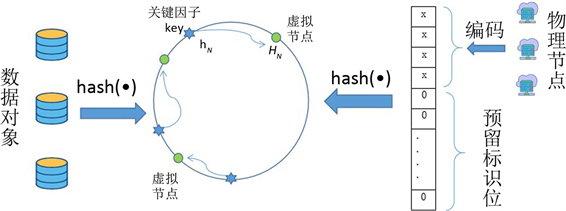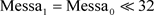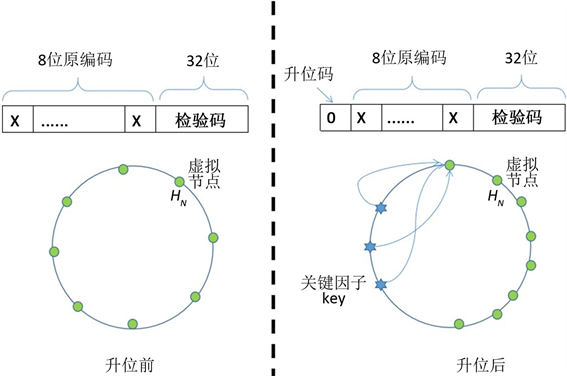# 基于一致性哈希算法的分布式数据库高效扩展方法Efficient Expansion Method for Distributed Database Based on Consistent Hashing Algorithm

• 全文下载: PDF(806KB)    PP.154-159   DOI: 10.12677/CSA.2020.101017
• 下载量: 89  浏览量: 136   国家科技经费支持

Under the big date background, distributed database became bigger during use. In the process of distributed database expansion, storage node’s hash value would be recomputed, and the data object would be migration, so a large amount of data will be transferred. An efficient expansion method for distributed database was adopted, by reserved identification bit and storage node’s code “High Bit Constant, Low Bit Set”. Contrast experiment shows that this method can avoid recomputed hash value of existing storage nodes as well as reduce data migration, and improve the efficiency of the distributed database expansion.

1. 引言

2. 预留分区的哈希空间构造Figure 1. A hash space with reserved partition’s identification bits

$C={x}_{M+N-1}\cdot {2}^{M+N-1}+\cdots +{x}_{M}\cdot {2}^{M}+0\cdot {2}^{M-1}+\cdots +0\cdot {2}^{0},\text{ }{x}_{i}\in \left\{0,1\right\}$ (1)

${C}_{N}={x}_{M+N-1}\cdot {2}^{M+N-1}+\cdots +{x}_{M-1}\cdot {2}^{M-1}+0\cdot {2}^{M-2}+\cdots +0\cdot {2}^{0},\text{ }{x}_{i}\in \left\{0,1\right\}$ (2)

${H}_{N}=\text{hash}\left({C}_{N}\right)$ (3)

3. 基于CRC32算法的哈希值生成

CRC32算法实际上是一种循环校验算法，原理是在一个P位二进制数据序列之后附加一个32-P位二进制检验码序列，从而构成一个总长为32位的二进制序列；附加检验码序列与原数据序列的内容之间存在着某种特定的关系。如果在传输过程中，数据序列中的某些位发生错误，这种特定关系就会被破坏，依据数据发送方和接收方原有的约定，接收方就可以发现这个错误。

$\begin{array}{c}\text{CRC}32={2}^{32}+{2}^{26}+{2}^{23}+{2}^{22}+{2}^{16}+{2}^{12}+{2}^{11}+{2}^{10}+{2}^{8}+{2}^{7}+{2}^{5}+{2}^{4}+{2}^{2}+{2}^{1}+1\\ =\text{0x04C11DB7}\end{array}$

1) 取校验多项式的阶次，CRC32算法的最高次项为232，所以其阶次为32；

2) 将原数据序列 ${\text{Messa}}_{0}$ 升位，得到3) 对 ${\text{Messa}}_{1}$ 进行模2除法，除数为CRC32，余数即CRC32的校验码：

${\text{CRC}}_{\text{Striing}}=\mathrm{mod}\left({\text{Messa}}_{\text{1}}/\text{CRC32}\right)$ (4)

4) 合成哈希值： $\text{hash_value}={\text{Messa}}_{1}+{\text{CRC}}_{\text{String}}$Figure 2. Realization block diagram of classical CRC algorithm

4. 分布式数据库扩展方法比较Figure 3. Position change of storage node’ hash value in hash space when expand digits at high positionFigure 4. Position change of storage node’ hash value in hash space when expand digits at low position

5. 结束语

2018 国家重点研发计划项目资助(项目编号 2018YFB1004604)；住房城乡建设部2016年科学技术项目资助(项目编号 2016-K3-008)。

  刘文洁, 李戬勃, 李战怀, 等. 一种面向金融应用的海量分布式关系数据库[J]. 华中科技大学学报(自然科学版), 2019, 47(2): 121-126.  门威, 邹香玲. 浅谈MapReduce与关系型数据库技术的融合[J]. 河北软件职业技术学院学报, 2017, 19(3): 10-13.  杨健, 刘方舟. 大数据背景下党建统计数据的存储电子化问题研究[J]. 云南民族大学学报(哲学社会科学版), 2019, 36(2): 26-30.  单维锋, 滕云田, 刘海军, 等. 大数据环境下地震观测数据存储方案研究[J]. 中国地震, 2019, 35(3): 558-564.  肖凌, 刘继红, 姚建初. 分布式数据库系统的研究与应用[J]. 计算机工程, 2001, 27(1): 33-35.  Karger, D., Lehman, E., Leighton, T., et al. (1997) Consistent Hashing and Random Trees: Distributed Caching Protocols for Relieving Hot Spots on the World Wide Web. Proceedings of the Twenty-Ninth Annual ACM Symposium on the Theory of Computing, El Paso, Texas, 4-6 May 1997, 654-663.  王康, 李东静, 陈海光. 分布式存储系统中改进的一致性哈希算法[J]. 计算机技术与发展, 2016, 26(7): 24-29.  陈凤, 蒙祖强. 基于哈希算法的异构多模态数据检索研究[J]. 计算机科学, 2019, 46(10): 49-54.